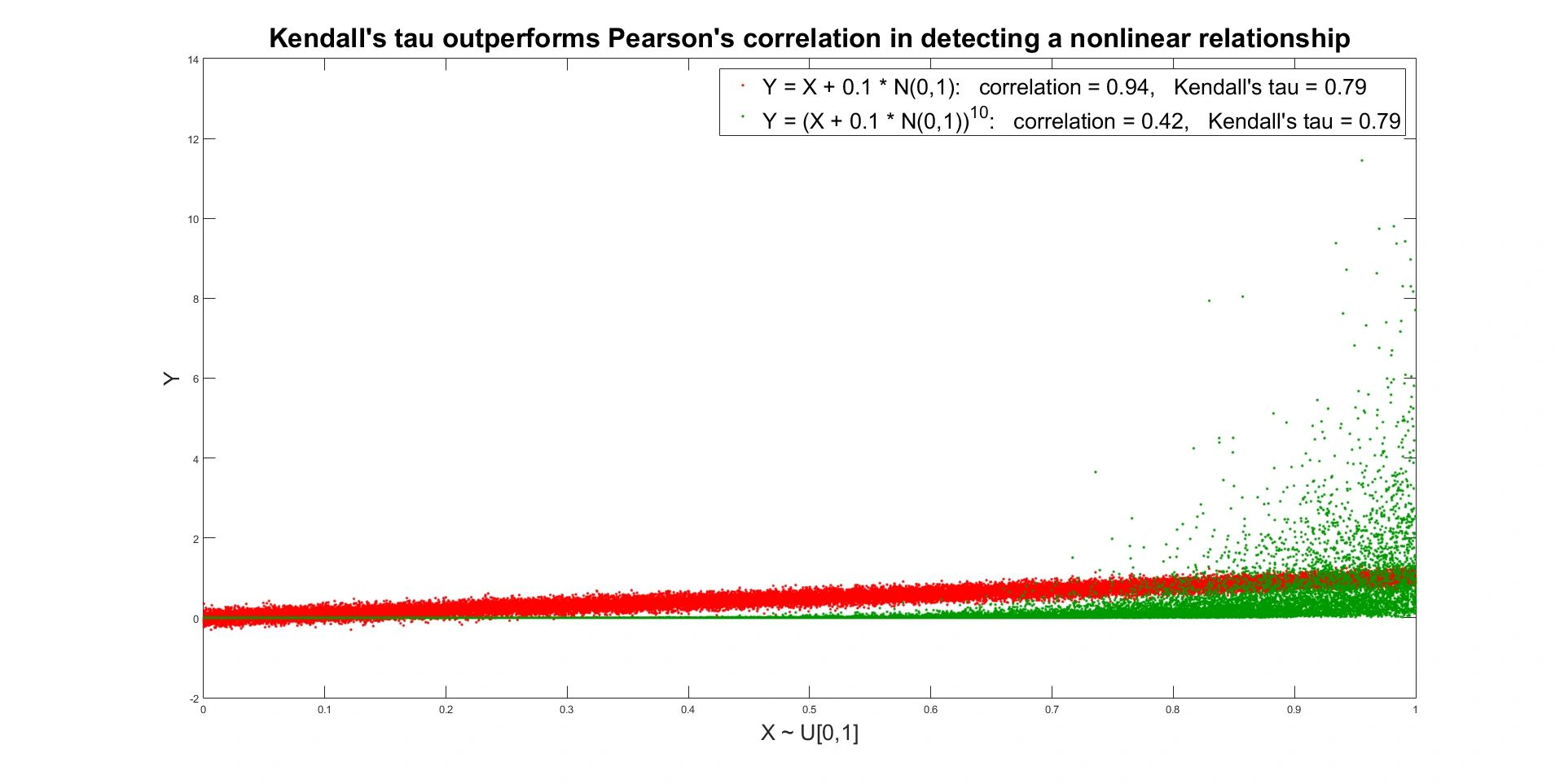Statistical & Financial Consulting by Stanford PhD
KENDALL'S TAU

Kendall's Tau (Kendall's Rank Correlation Coefficient) is a measure of nonlinear dependence between two random variables. If random variables and have joint distribution and random vectors and are independent realizations from that distribution, then Kendall's tau of and equals

If and have continuous marginal distributions then has the same units as Pearson's correlation. Just like Pearson's correlation it covers the whole range of [-1,1], but now -1 corresponds to a perfect negative relationship ( is any decreasing deterministic function of ) and 1 corresponds to a perfect positive relationship ( is any increasing deterministic function of ). When or has a discrete mass, interval [-1,1] is not covered fully. For example, if variable takes a given value with positive probability p, then with probability of at least p2 there is a tie: And so falls into interval [-1 + p2, 1 - p2] no matter what the bivariate relationship is. There are several proposals on how to adjust for ties, the most obvious one being to divide formula (1) by

Still, no single generalization has been widely accepted.

Note that definition (1) depends on ranks only. We only care if is bigger than the actual values being irrelevant. So Kendall's tau is invariant to any monotonically increasing nonlinear transformations of  and  If we raise to the third power Kendall's tau will stay the same. This is very important. Kendall's tau is naturally built to capture the strength of highly nonlinear relationships, where traditional linear association measures fail. The following graph illustrates the fact.Kendall's tau has direct relation to the copula function generated by random variables and

The copula function does not depend on marginal distributions and captures what happens to and if they are transformed into random variables uniformly distributed on [0,1]. The formula above signals once again that Kendall's tau does not depend on marginal distributions of and and is invariant to any monotonically increasing transformations of and

Several sample estimators have been developed. Let denote observations from the joint distribution of and Pairs and are called

concordant if their ranks agree: or

discordant if their ranks disagree: or

tied if or

Let
number of concordant pairs,

number of discordant pairs,

number of unique values in

number of unique values in

number of tied values in the i-th group of ties in

number of tied values in the j-th group of ties in

The estimators of are defined as

Estimators and make adjustments for ties and are suitable for all distributions. Estimator does not adjust for ties and is suitable only for continuous distributions measured with high precision. Each of the estimators is nonparametric in the sense that it makes little or no assumptions about the joint distribution of and In particular, no functional form is postulated for the conditional expectation of given and the conditional expectation of given For each of the estimators tests have been developed, telling us if equals 0. A typical test is based on a transformation of the estimator which is asymptotically normal (its distribution converges to a normal distribution when the sample size grows big).

KENDALL'S TAU REFERENCES

Nelsen, R. B. (2006). An Introduction to Copulas (2nd ed). New York: Springer.

Salvadori, G., De Michele, C., Kottegoda, N. T., & Rosso, R. (2007). Extremes in Nature: An Approach Using Copulas. Springer.

Gibbons, J. D., & Chakraborti, S. (2003). Nonparametric Statistical Inference (4th ed). New York: Marcel Dekker.

Nešlehová, J. (2007). On Rank Correlation Measures for Non-continuous Random Variables. Journal of Multivariate Analysis, Vol. 98, Issue 3, pp. 544-567.

Kendall, M. (1938). A New Measure of Rank Correlation. Biometrika, Vol. 30 (1–2), pp. 81–89.

BACK TO THE STATISTICAL ANALYSES DIRECTORY